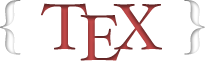## (Possibly) suspended users with the most rep.

0

(click on this box to dismiss)Q&A for users of TeX, LaTeX, ConTeXt, and related typesetting systems

``````WITH rawData(uid,userrep,uncapped,capped,accepts,bounties)
AS(
SELECT
tmp.[Reputation],
SUM(CASE
WHEN tmp.voteTotal >= 200 THEN 200
ELSE tmp.voteTotal END
SUM(tmp.acceptTotal) AS [Accepts],
SUM(tmp.bountyTotal) AS [Bounties]
--    CAST(100.0 * SUM(tmp.acceptTotal) / tmp.[Reputation] AS DECIMAL(20, 3)) AS [% from Accepts]
FROM
(SELECT
v.CreationDate,
COUNT(v.Id) AS votecount,
u.Reputation AS [Reputation],
SUM((CASE
WHEN v.CreationDate > p.CommunityOwnedDate THEN 0 -- After Community Wiki
WHEN VoteTypeId = 2 AND p.PostTypeId = 1 THEN 5     -- Upvoted question
WHEN VoteTypeId = 2 AND p.PostTypeId = 2 THEN 10    -- Upvoted answer
WHEN VoteTypeId = 3 THEN -2                       -- Downvote
ELSE 0
END)) AS voteTotal,
SUM((CASE
WHEN v.CreationDate > p.CommunityOwnedDate THEN 0 -- After Community Wiki
WHEN v.VoteTypeId = 1
and p.owneruserid <> q.owneruserid
ELSE 0
END)) AS acceptTotal,
SUM((CASE
WHEN v.VoteTypeId = 9 THEN BountyAmount           -- Earned Bounty
ELSE 0
END)) AS bountyTotal
JOIN VoteTypes vt ON vt.Id = v.VoteTypeId
JOIN Posts p ON p.Id = v.PostId
left outer join Posts q on q.id = p.parentid

WHERE
p.OwnerUserId is not null and
p.communityowneddate is null and
u.Reputation = 1
GROUP BY
u.Reputation,
v.CreationDate,
p.OwnerUserId
) AS tmp

GROUP BY
tmp.[Reputation]
)

SELECT TOP 1000
userrep AS [Reputation],
capped + accepts + bounties AS [DE Recalced Rep],Hold tight while we fetch your results